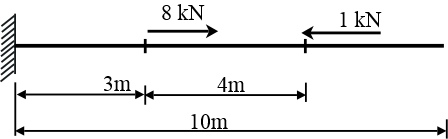# Consider a bar with a length of 10m with a constant circular cross-section with a radius of 19mm....

## Question:

Consider a bar with a length of 10m with a constant circular cross-section with a radius of 19mm. The bar consists of an aluminum alloy with a Young's modulus of 70GPa and a Poisson Ratio of 0.3. It is rigidly fixed at its left edge and subject to two point forces: one force of 8 kN at the cross-section 3m from the fixed end and another force of 1 kN, 7m from the fixed end. Use the method of superposition to determine the distribution of all the strains in this bar.## Poisson's Ratio:

Poisson's ratio is used to find out lateral strain when longitudinal strain is given or lateral strain when longitudinal strain is given. It is a unitless quantity. It is indeed the ratio between the lateral strain and the longitudinal strain.

Given Data

• The load at point B is: {eq}{F_B} = 8\;{\rm{kN}} = 8000\;{\rm{N}} {/eq}
• The load at point C is: {eq}{F_C} = 1\;{\rm{kN}} =...

Become a Study.com member to unlock this answer! Create your account